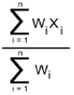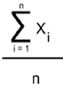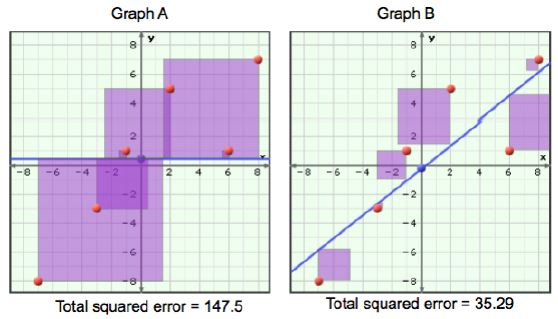Name:    IDC 4UB - Building Financial Security

Multiple Choice
Identify the choice that best completes the statement or answers the question.

1.

Which of the following is NOT a measure of dispersion in a set of data?
 a. mean b. histogram c. variance d. standard deviation

2.

The formula below will determine thea. population mean b. sample mean c. mode d. median e. grouped data average f. weighted average

3.

This formula will calculate thea. grouped data median b. mode c. median d. mean e. weighted average

4.

In the formula for standard deviation (for a sample of data) below, the reason for ∑(x - µ)2 in the formula is:a. to find all the differences in the data b. to sum all the squares of error in the data set c. finding all the deviation d. to determine the standard deviation e. to calculate the mean f. to measure volatility

5.

Which expression best defines standard deviation?
 a. Volatility. b. The total error in the data set c. The sum of all squares of error in the data set d. How much a random data point will vary from µ on average e. The error in the data set using the average to find it

6.

Below are the investment weightings of each kind of investment for Bob, Pavneet, and Jose.

 Investments Return% Bob’s weights Pavneet’s weights Jose’s weights Cash 0 10 10 25 Bond fund 10 20 20 40 Income fund 15 20 50 30 Growth fund -5 50 20 5

Based on the information in the chart above, which investment portfolio will have the highest investment growth?
 a. Bob b. Pavneet c. Jose d. None of them

7.

Why is mean, median, or mode never enough to describe a data set on their own?
 a. You don’t know what the min and max values are b. You don’t know how the individual data points are distributed throughout the range c. You can’t tell if there is more than one central tendency to the data d. all of these answers are correct.

8.

One of the best and most-used tools to visualize one variable statistics that eliminates many of the limitations of measures of centre is:
 a. Scatter plot b. Bar graph c. Line graph d. Histogram e. Pie grapm

9.

What is the independent variable in a correlational study of amounts of sunlight and the heights of tomato plants?
 a. the types of tomato plants b. the heights of the tomato plants c. the angle of the sun d. the numbers of hours of sunlight

10.

Discuss the observation that “Drivers of red cars are twice as likely to be involved in an accident as drivers of blue cars.” Does this imply that driving a red car “causes” drivers to have an accident? What is the relationship here?
 a. causation b. common cause c. coincidence d. none of the above

11.

A higher number of ice cream sales corresponds to a higher number of shark attacks on swimmers.
 a. causation b. common cause c. coincidence d. none of the above

12.

Which of the following is the dependent variable?
 a. hours of basketball practice b. free-throw success rate c. could be either

13.

Which of the following is the dependent variable?
 a. running speed b. pulse rate c. could be either

14.

Which set of data would probably show a strong positive linear correlation?
 a. marks on a history test and the heights of the students b. the number of defective light bulbs produced and the time of the day when they were manufactured c. the colour of cars sold and the annual income of the car buyers d. the hight of the corn in a field and the amount of precipitation during the growing season

15.

Which set of data would probably show a strong negative linear correlation?
 a. resale values of computers and their ages b. heights of volleyball players can jump and the strength of their leg muscles c. numbers of people at a water park and the air temperature d. scores on a mathematics test and the number of hours spent studying for it

16.

If the rate of ozone depletion and the temperature were shown to be negatively correlated, then
 a. a low rate of depletion would occur at lower temperatures b. a high rate of depletion would occur at higher temperatures c. a low rate of depletion would occur at higher temperatures d. a high rate of depletion would occur at lower temperatures e. (a) and (b) are correct f. (c) and (d) are correct g. None are correct

17.

A set of data with a correlation coefficient of –0.55 has a
 a. strong negative linear correlation b. moderate negative linear correlation c. weak negative linear correlation d. little or no linear correlation

18.

The correlation coefficient for weed growth in a lake and temperature was found to be 0.915. The scatter plot for the data would have
 a. an array of dots with no discernible pattern to them b. dots clustered in a linear fashion sloping up to the left c. dots tightly clustered in a linear fashion  sloping up to the right d. a cluster of dots in the middle of the graph

19.

For the line of best fit in the least-squares method,
 a. the sum of the squares of the residuals (squared error) has the greatest possible value b. the sum of the squares of the residuals (squared error) has the least possible value c. the sum of the residuals is equal to one d. both (b) and (c)

20.

An outlier is a data point that
 a. should sometimes be left out of a statistical analysis b. may be an abnormal result c. may significantly affect the calculation of the correlation coefficient d. all of these are correct

21.

The coefficient of determination (the goodness of fit), r2, indicates
 a. the linear relationship between two variables b. the slope of the line of best fit (i.e. the regression line) c. how closely the data fit a defined curve d. the percentage of squared (residual) error removed by regression

22.

Observe the graphs below.The y-values are daily maximum temperatures, their average is 0.5 degrees celcius. The purple squares in graph A indicate the squared error from using a simple average temperature (the line is y=0.5) to predict the value of a single data point. In graph B  the squared error is from using a model of linear regression to predict temperature. Thus, the value of the coefficient of determination (the goodness of fit), r2 for the linear model in graph B is likely:

 a. 0.21 b. zero c. 0.97 d. 0.76 e. 61.0

23.

The population of certain species of animals decreases as logging in wilderness areas increases is most likely an example of a
 a. causal (i.e. cause-and-effect) relationship b. common cause relationship (i.e. both are related to some third unknown variable) c. coincidental relationship

24.

The price of bread and canola oil both increase sharply after the prairies experience a drought during the growing season is most likely an example of a
 a. causal (i.e. cause-and-effect) relationship b. common cause relationship (i.e. both are related to some third unknown variable) c. coincidental relationship

25.

The current world price of crude oil increases as the price of gasoline at the pump increase is most likely an example of a
 a. causal (i.e. cause-and-effect) relationship b. common cause relationship (i.e. both are related to some third unknown variable) c. coincidental relationship

26.

Does the slope of a line of regression (line of best fit) tell you anything (on its own) about the relationship of the two variables?
 a. yes, lots b. almost nothing c. nothing d. none of these answers is correct

The coach of the Statsville football team wants to determine if there is a relationship between how fast players can run 60 m and how far they can throw the football. The results for the Statsville players are graphed in the scatterplot below.27.

Based on the scatter plot, are there any data points that could be identified as outliers?
 a. Yes, one. b. Yes, two. c. No, none d. There are several.

28.

If the regression line for this scatter plot is: Throwing Distance = -3(Sprint Time) + 50, then use the model to predict the throwing distance of an athlete who can sprint 60m in 5 seconds.
 a. 68.6 m b. 50 m c. 40 m d. 35 m e. The answer is not on this list

29.

Based on the scatter plot (ignoring any outliers), what type of relationship exists between the two variables?
 a. Weak positive relationship b. Strong positive relationship c. Weak negative relationship d. Strong negative relationship e. No relationship

30.

Which one of the following images showing relationships on a scatter plot would have a correlation coefficient of r = 0.6?a. graph A b. graph B c. graph C d. graph D e. all of them f. none of them

31.

Why are the upper and lower limits of bollinger bands or a regression channel defined by ± 2 standard deviations?
 a. This will encompass all the data points that can occur b. They define the upper and lower limits of the trend c. Because one expects only 1 in 20 data points to exceed these limits d. None of the above

32.

Why is ß represented by the slope of a least-squares line when you plot a security’s return against the market return on a scatter plot?
 a. Because it’s a mathematically precise way of finding the trend line. b. It’s a more accurate way of finding standard deviation c. Because slope measures the magnitude of y’s change to x’s change d. Because you need to know R2 to use it.

33.

Which definition does not represent expected value?
 a. A weighted average b. The value outcome that is most likely c. The probability of a value outcome. d. The per trial value you would expect to get

34.

What are the only two values needed to describe a normal probability distribution?
 a. Beta and standard deviation b. Standard deviation and median c. Range and average d. Average and standard deviation e. Mean and probability

35.

Which of the following coloured lines would correspond to a normal distribution with standard deviation = 0.5 and 0.7 respectively?a. Blue and green b. Red and gold c. Blue and gold d. Green and red